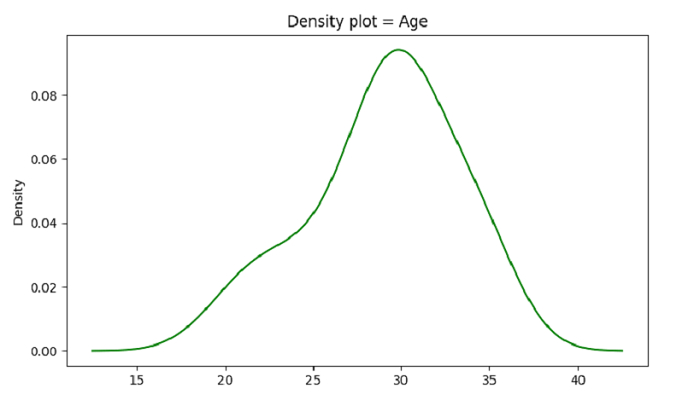# Python - Density Plots with Pandas for a specific attribute

We will use plot.density() to density plot on a Dataset in the form of a csv file. Let’s say the following is our dataset − Cricketers2.csv

At first, import the required libraries −

import pandas as pd
import matplotlib.pyplot as plt

Load data from a CSV file into a Pandas DataFrame −

dataFrame = pd.read_csv("C:\Users\amit_\Desktop\Cricketers2.csv")


Plotting the density plot. Attribute considered is "Age" −

dataFrame.Age.plot.density(color='green')

## Example

Following is the complete code −

import pandas as pd
import matplotlib.pyplot as plt

# Load data from a CSV file into a Pandas DataFrame

# plotting the density plot
# attribute considered is "Age"
dataFrame.Age.plot.density(color='green')
plt.title('Density plot = Age')
plt.show()

## Output

This will produce the following output −